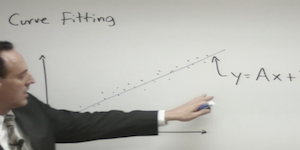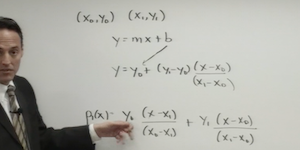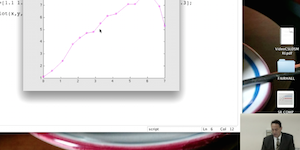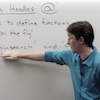# Beginning Scientific Computing

## Curve Fitting

### Lecture 1### [ view ]

LEAST-SQUARE FITTING METHODS: The basic theory of curve fitting and least-square error is developed.

MATLAB COMMANDS

`n/a`

### Lecture 2### [ view ]

POLYNOMIAL FITS AND SPLINES: Polynomial fitting of the data, via Lagrange polynomials, can also be considered as the fit curves go through all data points. Spline technology is developed to circumvent polynomial wiggle.

MATLAB COMMANDS

`n/a`

### Lecture 3### [ view ]

DATA FITTING WITH MATLAB: We develop a MATLAB code that implements all the theoretical methods considered for curve fitting: least-square fits, polynomial fits and splines.

MATLAB COMMANDS

`POLYFIT POLYVAL SPLINE INTERP1`

### Supplementary VideosView
Function Handles in MATLAB
Code: file.m
© 2015 kutz